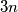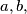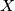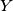### IMO Shortlist 2017 problem C2

Kvaliteta:
Avg: 0.0
Težina:
Avg: 6.0

Let$n$ be a positive integer. Define a chameleon to be any sequence of$3n$ letters, with exactly$n$ occurrences of each of the letters$a, b,$ and$c$. Define a swap to be the transposition of two adjacent letters in a chameleon. Prove that for any chameleon$X$ , there exists a chameleon$Y$ such that$X$ cannot be changed to$Y$ using fewer than$3n^2/2$ swaps.

Source: https://www.imo-official.org/problems/IMO2017SL.pdf